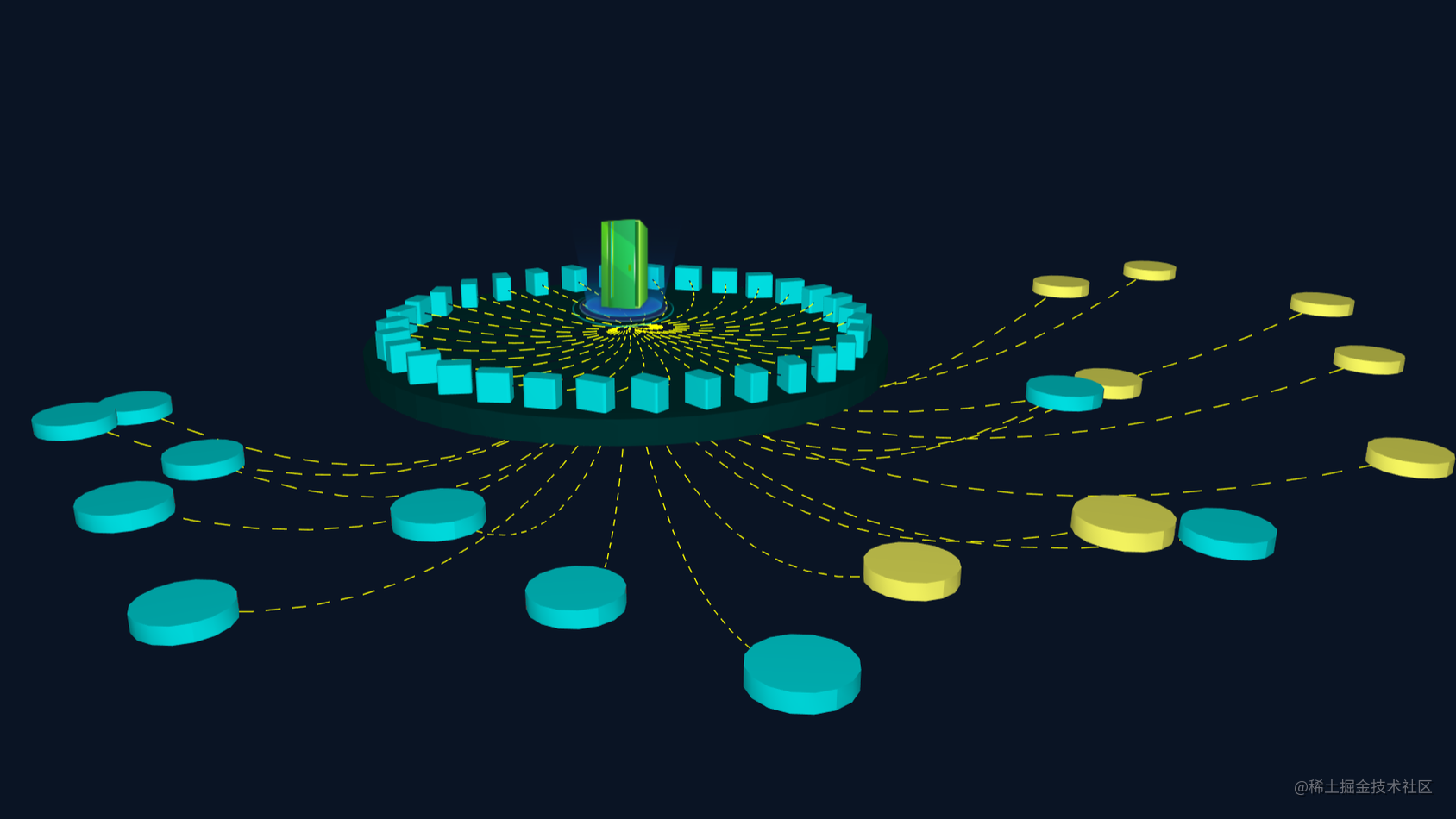# 基于 HTML5 WebGL 的 3D 网络拓扑图1、准备工作：

3D 和 2D 的 API 的设计上保持了很多一致性，3D 视图组件是 ht.graph3d.Graph3dView， 2D 视图组件是 ht.graph.GraphView，两者可共享同一数据模型 DataModel。在 HT 中，为了让了获得接近真实三维物体的视觉效果，我们通过透视投影使得远的对象变小，近的对象变大，平行线会出现先交等更接近人眼观察的视觉效果：• getEye() | setEye([x, y, z])，决定眼睛（或 Camera）所在位置，默认值为 [0, 300, 1000]；
• getCenter() | setCenter([x, y, z])，决定目标中心点（或 Target）所在位置，默认值为 [0, 0, 0]；

``````dataModel = new ht.DataModel();
g3d = new ht.graph3d.Graph3dView(dataModel);
g3d.setEye(1800, 800, 1000);
g3d.setCenter(0, 100, 0);
g3d.setDashDisabled(false);
g3d.getView().style.background = 'rgb(10, 20, 36)';

2、创建设备：

``````// 注册图片
ht.Default.setImage('server', 'server.png');

var server = new ht.Node();
server.s3(0, 0, 0);
server.p3(0, 60, 0);
position: 0,
width: 200,
autorotate: true,
transparent: true,
height: 200,
names: ['server']
});

``````var desktop = new ht.Node();
desktop.s({
'3d.selectable': false,
'shape3d': ht.Default.createRingModel([
0, 40,
450, 40,
450, 0,
0, 40
], null, 20, false, false, 50),
'shape3d.color': '#003333'
});
desktop.s3(1, 1, 1);

``````var count = 32;
index = count/2;
for (var i =  1; i <= count/2; i++) {
var device1_angle1 = Math.PI * 2 * (index - i) / count;
device1_angle2 = Math.PI * 2 * (index + i) / count;
device1_angle3 = Math.PI * 2 * index / count;

var device1_1 = createDevice(device1_angle1, radius, 60);

layoutDevice1(device1_1, device1_angle1);
var device1_edge1 = createEdge(device1_1, server, 'line1');
device1_edge1.s({'shape3d.color': 'rgb(205, 211, 34)'});

layoutDevice1(device1_2, device1_angle2);
var device1_edge2 = createEdge(device1_2, server, 'line1');
device1_edge2.s({'shape3d.color': 'rgb(205, 211, 34)'});

layoutDevice1(device1_3, device1_angle3);
var device1_edge3 = createEdge(device1_3, server, 'line1');
device1_edge3.s({'shape3d.color': 'rgb(205, 211, 34)'});
}复制代码``````

``````function createDevice (angle, x, y) {
var node = new ht.Node();
cos = Math.cos(angle);
sin = Math.sin(angle);
node.p3(x*sin, y, x*cos);
return node;
}复制代码``````

``````var num = 18;
var h = [800, 900, 1000, 1100, 1200];
var v = [40, 60, 80, 100];
var colors = ['#fcfc63', '#00E1E4'];
for (var j = 0; j < num; j++) {
var device2_angle = Math.PI * j / num;
var device2 = createDevice(device2_angle,
h[Math.floor(Math.random()*5)], v[Math.floor(Math.random()*4)]);
device2.s3(100, 20, 100);
device2.s({
'shape3d': 'cylinder',
'shape3d.color': colors[Math.floor(Math.random()*2)]
});
var device2_edge = createEdge(device2, desktop , 'line2');
device2_edge.s({'shape3d.color': 'rgb(0, 203, 94)'});

}复制代码``````

3、连线

HT for Web 提供了默认的直线和多点的连线类型能满足大部分基本拓扑图形应用，但在这里我们需要根据实际需求绘制曲线，所以，需要用到自定义连线类型，详情看 HT for Web 连线类型手册：

• type：字符串类型的连线类型，对应 style 的 edge.type 属性；
• func：函数类型，根据传入参数（edge，gap，graphView，sameSourceWithFirstEdge）返回连线走向信息

edge：当前连线对象；

gap：多条连线成捆时，本连线对象对应中心连线的间距；

graphView：当前对应拓扑组件对象；

sameSourceWithFirstEdge：boolean 类型，改连线是否与同组的第一条同源；

1、moveTo，占用 1 个点信息；

2、lineTo，占用 1 个点信息；

4、bezierCurveTo，占用 3 个点信息；

5、closePath，不占用点信息；

• mutual：该参数决定连线是否影响起始或结束节点上的所有连线，默认为 false 代表只影响同 source 和 target 的

EdgeGroup 中的连线，HT 预定义的连线类型中，后缀为 2 的类型都是 mutural 为 true 的复杂连线类型。

``````ht.Default.setEdgeType('line1', function(edge){
var sourcePoint1 = edge.getSourceAgent().getPosition(),
targetPoint1 = edge.getTargetAgent().getPosition(),
points1 = new ht.List();
x: (sourcePoint1.x + targetPoint1.x)/2 + 200,
e: sourcePoint1.e,
y: (sourcePoint1.y + targetPoint1.y)/2
});
return {
points: points1,
segments: new ht.List([1, 3])
};
});
ht.Default.setEdgeType('line2', function(edge){
var sourcePoint = edge.getSourceAgent().getPosition(),
targetPoint = edge.getTargetAgent().getPosition(),
points = new ht.List();
x: (sourcePoint.x + targetPoint.x)/2,
e: ((sourcePoint.e + targetPoint.e)/2 || 0) - 300,
y: (sourcePoint.y + targetPoint.y)/2
});
x: targetPoint.x,
e: targetPoint.e -80,
y: targetPoint.y
});
return {
points: points,
segments: new ht.List([1, 3])
};
});复制代码``````

``````function createEdge (source, target , type) {
var edge = new ht.Edge(source, target);
edge.s({
'edge.color': 'yellow',
'edge.dash': true,
'edge.dash.3d': true,
'edge.dash.width': 4,
'edge.type': type,
'edge.dash.color': 'rgb(10, 20, 36)',
'edge.dash.pattern': [20, 25]
});
edge.a({
'flow.enabled': true,
'flow.direction': -1,
'flow.step': 4
});
return edge;
}复制代码``````

``````flowTask = {
interval: 50,
action: function(data){
if(data.a('flow.enabled')){
var offset = data.s('edge.dash.offset') + data.a('flow.step') * data.a('flow.direction');
data.s('edge.dash.offset', offset);
}
}
};